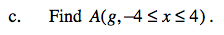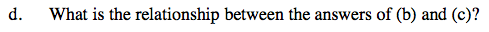### Home > CALC > Chapter 1 > Lesson 1.1.1 > Problem1-5

1-5.
1. Quickly sketch the function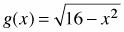. Homework Help ✎

1. State the domain and range of g(x).

2. Use geometry to find A(g, 0 ≤ x ≤ 4).

3. Find A(g, − 4 ≤ x ≤ 4).

4. What is the relationship between the answers of (b) and (c)?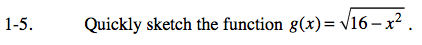You should recognize this function as a semicircle with radius 4, centered at the origin.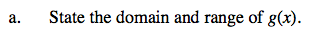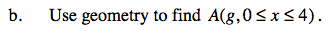$\text{On the graph of }g(x),\text{ shade the region }A(g,0\leq x\leq 4).\text{ Observe that this area is }\frac{1}{4}\text{ the area of a }$

$\text{ complete circle, and }\frac{1}{2}\text{ the area of the semicircle, }g(x).$